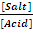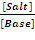## Sunday, March 4, 2012

### Verification of the Henderson-Hasseblach Equation Using Buffer Solution

Objectives:

1. To study the validity of Henderson-Hasseblach equation to a buffer system

2. To determine the buffer capacity of a buffer solution

Introduction:

Buffers are solutions that maintain a relatively constant pH when an acid or a base is added into a buffer solution. They protect other molecules in solution from the effects of the added acid or base. Buffer solution contains either a weak acid (HA) and its conjugate base (A-) or a weak base (B) and its conjugate acid (BH+). They are extremely important for the proper functioning of biological systems such as blood, which is a natural example of buffer solution.

An acid is defined as a substance that can dissociate in water to produce hydrogen ions, H+ while a base is a substance that can dissolve in water to produce hydroxide ions, OH-. The acid is categorized into two groups which are strong acid and weak acid. Strong acid is the acid that dissolves completely in water to produce a lot of H+ ions whereas weak acid is the acid that ionizes partially in water to produce less H+ ions.

An important characteristic of weak acids and weak bases is their ability to form buffer systems. The action of a buffer is caused by the presence of both the weak acid and its anion or the weak base and its cation. These are known as conjugate acid-base pair. If strong base is added into a weak base, it will dissociate into OH- and conjugate acid. The conjugate acid produced will neutralize the base added and hence control it pH. On the other hand, if string acid is added, the weak base will react with the strong acid by neutralizing it. So, the pH of the solution can be maintained. This is same applied by the acid buffer system with H+ ion and its conjugate base.

Consider a sodium acetate-acetic acid buffer system in which containing the same amounts of acetic acid (CH3COOH) and its conjugate base, acetate ion (CH3COO-). When the addition of a strong acid hydronium ion (H3O+) to the solution. The acetate ion (base form) in the buffer reacts with the hydornium ion to neutralize it.

In the same way, the addition of the strong base provide hydroxide ion, OH- to the solution, then the acetic acid will reacts with this ion and neutralize it.

The amount of strong acid or base can be added to a given volume of a buffer system without a significant change in pH in +/- 1.0 unit. This is known as the buffer capacity of a buffer system.

The strength of acid is depends on the degree of H+ ionization in the water. For base, its strength is depends on the degree of OH- ionization in the water.

The magnitude of the equilibrium constant for an ionization reaction can be used to determine the relative strengths of acids and bases. The equilibrium constant for acid dissociation is

The equilibrium constant for base dissociation is

In order to obtain Henderson Hasselbalch equation, rearrange the following equation:

Buffer capacity of a buffered solution is defined as in terms of the amount of protons or hydroxide ions it can absorb without a significant change in buffer. A buffer with a large capacity contains large concentration of the buffering components and thus can absorb a relatively large amount of protons or hydroxide ions and show little pH change. The pH of a buffer system is determined by the ratio [A-]/[HA]. The capacity of a buffer system is determined by the magnitude of [A-] and [HA]. Buffer capacity is also depends on the concentration of the solution. The greater the concentration of a weak acid and its conjugate base, the greater the buffer capacity. Buffer capacity may expressed as

A buffer solution has the maximum buffer capacity when the ratioor= 1 for acidic buffer and basic buffer respectively. In such a solution pH = pKa for acidic buffers and pOH= pKb for basic buffers. A solution can act as buffer only if the ratio of salt to acid or base is between 0.1 and 10. Thus, the pH of an acidic buffer can have the range from pKa - 1 or pKa + 1. Similarly, a basic buffer can act as buffer in the pOH range from pKb – 1 to pKb + 1.

Materials: standard buffer solution (pH= 4.00), 1 M aqueous sodium hydroxide, 1 M aqueous acetic acid

Apparatus: burettes, beaker (100cm3), pH meter

Procedure:

1. The pH meter is calibrated by standard buffer solution, pH=4.00.

2. 50cm3 of the 1 M acetic acid and 1 M sodium hydroxide are pipetted into a 100cm3 beaker.

3. The solution is mixed thoroughly and pH of the solution is measured.

4. The pH measurement is repeated for further 1 cm3 addition each up to a total of 5cm3 of 1 M sodium hydroxide.

5. The pH of the solution is measured for further 5cm3 additions each up to a total of 45cm3 of 1 M sodium hydroxide.

6. The pH measurement is repeated for further 1 cm3 addition each up to a total of 50cm3 of 1 M sodium hydroxide.

Result and calculation:

Table 1 The average pH value of buffer solution
 Volume of aqueous 1 M CH3COOH (X) ,cm3 Volume of aqueous 1 M NaOH (Y), cm3 pH [ Salt ] / [ Acid ] = y/(x-y) Log10 ( [ Salt ] / [ Acid ] 50 1 2.97 0.0204 -1.690 50 2 3.26 0.0417 -1.380 50 3 3.45 0.0638 -1.195 50 4 3.58 0.0870 -1.060 50 5 3.69 0.1111 -0.954 50 10 4.06 0.2500 -0.602 50 15 4.32 0.4286 -0.368 50 20 4.54 0.6667 -0.176 50 25 4.74 1.0000 0.000 50 30 4.95 1.5000 0.176 50 35 5.21 2.3333 0.368 50 40 5.65 4.0000 0.602 50 45 10.03 9.0000 0.954 50 46 11.76 11.5000 1.061 50 47 12.03 15.6667 1.195 50 48 12.19 24.0000 1.380 50 49 12.29 49.0000 1.690 50 50 12.37 - -

Turning point at pH

= (5.65+10.03)/ 2

= 7.84

#### 1 comment:

1.How to calculate the buffer capacity?